# Ncert Solutions for Class 6 Mathematics Decimals Exercise 8.2,8.3,8.4

In this page we have Ncert Solutions for Class 6 Mathematics Decimals Exercise 8.2,8.3,8.4. Hope you like them and do not forget to like , social share and comment at the end of the page.

## Exercise 8.2

Question 1
Express as rupees using decimals.
(a) 5 paise
(b) 75 paise
(c) 20 paise
(d) 50 rupees 90 paise
(e) 725 paise
We know that 100 paise = Rs 1
Therefore, 1 paise = Rs 1/100
(a) 5 p = 5/100=Rs .05
(b) 75 p = 75/100= Rs .75
(c) 20 p = 20/100= Rs .2
(d) 50 rupees 90 paise = 50 + 90/100= Rs 50.9
(e) 725 paise= 725/100 = Rs 7.25

Question 2
Express as metres using decimals.
(a) 15 cm
(b) 6 cm
(c) 2 m 45 cm
(d) 9 m 7 cm
(e) 419 cm
1 m= 100 cm so 1 cm =1/100 m
(a) 15 cm= 15/100 m =.15 m
(b) 6 cm= 6/100 m =.06m
(c) 2 m 45 cm = (2+ 45/100) m= 2.45 m
(d) 9 m 7 cm = (9+ 7/100) m= 9.07 m
(e) 419 cm= 4.19 m

Question 3
Express as km using decimals.
(a) 8 m
(b) 88 m
(c) 8888 m
(d) 70 km 5 m
1km =1000m
1m =1/1000 km
(a) 8 m = 8/1000 km=.008 km
(b) 88 m = 88/1000 km=.088 km
(c) 8888 m = 8888/1000 km=8.888 km
(d) 70 km 5m = (70 + 5/1000) km=70.005 km

Question 4
Express as kg using decimals.
(a) 2 g
(b) 100 g
(c) 3750 g
(d) 5 kg 8 g
(e) 26 kg 50 g
1kg =1000g
1g =1/1000 kg
(a) 2 g = 2/1000 kg=.002 kg
(b) 100 g = 100/1000 kg=.1 kg
(c) 3750 g = 3750/1000 kg=3.75 kg
(d) 5kg 8g = (5+ 8/1000) kg=5.008 kg
(e) 26kg 50 g = (26+ 50/1000) kg=26.50 kg

## Exercise 8.3

Question 1
Find the sum in each of the following:
(a) $0.007 + 8.5 + 30.08$
(b) $15 + 0.632 + 13.8$
(c) $27.076 + 0.55 + 0.004$
(d) $25.65 + 9.005 + 3.7$
(e) $0.75 + 10.425 + 2$
(f) $280.69 + 25.2 + 38$
(a) $0.007 + 8.5 + 30.08$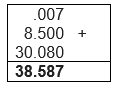(b) $15 + 0.632 + 13.8$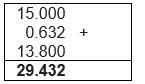Similarly
(c) $27.076 + 0.55 + 0.004$
=27.630
(d) $25.65 + 9.005 + 3.7$
=38.355
(e) $0.75 + 10.425 + 2$
=13.175
(f) $280.69 + 25.2 + 38$
=343.890

Question 2
Rashid spent Rs 35.75 for Math’s book and Rs 32.60 for Science book. Find the total amount spent by Rashid.
Amount spent for Math’s book = Rs. 35.75
Amount spent for Science book = Rs. 32.60
Total amount spent for the books by Rashid = 35.75 + 32.60=68.35
So, Rashid spent Rs. 68.35 for the books.

Question 3
Radhika’s mother gave her Rs 10.50 and her father gave her Rs 15.80, find the total amount given to Radhika by the parents?
Money given by mother to Radhika = Rs. 10.50
Money given by father to Radhika = Rs. 15.80
Doing the addition similarly as given above
Total amount=26.30

Question 4
Nasreen bought 3 m 20 cm cloth for her shirt and 2 m 5 cm cloth for her trouser. Find the total length of cloth bought by her.
We know that 1 m=100 cm and 1 cm = 1/100 m
Length of the cloth purchased for shirt = 3 m 20 cm =3m + 20/100 m= 3.2 m
Length of the cloth purchased for trouser = 2 m 5 cm
=2m + 5/100m = 2.05 m
Total length of the cloth purchased
=3.20 + 2.05
Total length of the cloth purchased = 5.25 m

Question 5
Naresh walked 2 km 35 m in the morning and 1 km 7 m in the evening. How much distance did he walk in all?
We know that 1 km=1000 m and 1 m = 1/1000 km
Distance walked by Naresh in the morning = 2 km 35 m
= 2 km + 35/1000 km= 2.035 km
Distance covered by Naresh in the evening = 1 km 7 m
= 1 km + 7/1000 km= 1.007 km
Distance Naresh walked in all = $(2.035 + 1.007)$ km
=3.042 km

Question 6
Sunita travelled 15 km 268 m by bus, 7 km 7 m by car and 500 m on foot in order to reach her school. How far is her school from her residence?
We know that 1 km=1000 m and 1 m = 1/1000 km
Distance travelled by Sunita in bus = 15 km 268 m
= 15 km  + 268/1000 km= 15.268 km
Distance travelled by Sunita in car = 7 km 7 m
=7 km + 7/1000 km= 7.007 km
Distance travelled by Sunita on foot = 500 m = 0.5 km
Total distance of school from residence =$15.268 + 7.007 +0.5 =22.775$ km

Question 7
Ravi purchased 5 kg 400 g rice, 2 kg 20 g sugar and 10 kg 850 g flour. Find the total weight of his purchases.
Amount of rice = 5 kg 400 g = 5.400 kg
Amount of sugar = 2 kg 20 g = 2.020 kg
Amount of flour = 10 kg 850 g = 10.850 kg
Total weight of the purchases =5.400 kg + 2.020 kg + 10.850 kg
=18.270 kg
Total amount of purchase = 18.270 kg

## Exercise 8.4

Question 1
Subtract:
(a) Rs 18.25 from Rs 20.75
(b) 202.54 m from 250 m
(c) Rs 5.36 from Rs 8.40
(d) 2.051 km from 5.206 km
(e) 0.314 kg from 2.107 kg

(a)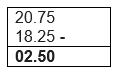(b)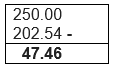(c)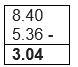(d)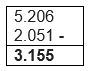(e)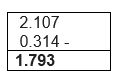Question 2
Find the value of:
(a) $9.756 - 6.28$
(b) $21.05 - 15.27$
(c) $18.5 - 6.79$
(d) $11.6 - 9.847$
Solving similarly as above
(a) $9.756 - 6.28 =3.476$
(b) $21.05 - 15.27=5.78$
(c) $18.5 - 6.79=11.71$
(d) $11.6 - 9.847=1.753$

Question 3
Raju bought a book for Rs 35.65. He gave Rs 50 to the shopkeeper. How much money did he get back from the shopkeeper?
Cost of the book Raju purchased = Rs. 35.65
Money paid by Raju to the shopkeeper = Rs. 50
Remaining = Rs. 50 – Rs. 35.65
Solving the subtraction similarly as given above
= Rs 14.35

Question 4
Rani had Rs 18.50. She bought one ice-cream for Rs 11.75. How much money does she have now?
Money initially with Rani = Rs. 18.50
Amount Rani spent on an ice-cream = Rs. 11.75
Remaining Money with Rani = $18.50 - 11.75$
Solving the subtraction similarly as given above
= Rs 6.75

Question 5
Tina had 20 m 5 cm long cloth. She cuts 4 m 50 cm length of cloth from this for making a curtain. How much cloth is left with her?
Length of the cloth which Tina had = 20 m 5 cm = 20.05 m
Length of the cloth which Tina cuts = 4 m 50 cm = 4.50 m
Length of the cloth left with her = 20.05 m – 4.50 m
=15.55 m

Question 6
Namita travels 20 km 50 m every day. Out of this, she travels 10 km 200 m by bus and the rest by auto. How much distance does she travel by auto?
1 Km =1000m and 1 m=1/1000 km Total distance Namita travelled every day is 20 km 50 m = 20.050 km
Distance travelled in bus is 10 km 200 m = 10.200 km
Distance travelled in auto= Total distance travelled - distance travelled in Bus
$=20.050 - 10.200 =9.850 km$

Question 7
Aakash bought vegetables weighing 10 kg. Out of this, 3 kg 500 g is onions, 2 kg 75 g is tomatoes and the rest is potatoes. What is the weight of the potatoes?
We know that 1 kg=1000g and 1 g= 1/1000 kg
Given
Total weight of the vegetables purchased by Aakash = 10 kg
Weight of onions purchased = 3 kg 500 g = 3.500 kg
Weight of tomatoes of Purchased = 2 kg 75 g = 2.075 kg
Total Weight of onions and tomatoes = 3.500 + 2.075 kg
=5.575 kg
Weight of potatoes = Total Weight of the vegetables – weight of onions and tomatoes

$=10- 5.575 =4.425$ kg### Practice Question

Question 1 What is $\frac {1}{2} + \frac {3}{4}$ ?
A)$\frac {5}{4}$
B)$\frac {1}{4}$
C)$1$
D)$\frac {4}{5}$
Question 2 Pinhole camera produces an ?
A)An erect and small image
B)an Inverted and small image
C)An inverted and enlarged image
D)None of the above